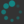## Exercises

In this problem set, we will use angle brackets to denote points, e.g. $$\point{1\\0},$$ and square brackets to denote vectors, e.g. $\vector{3\\2}.$ This is not standard notation. Typically both are denoted by square brackets (or parentheses), and distinguished by context (if at all).

1. Compute the following expressions if they make sense, or answer "meaningless" if they do not make sense.
1. $$\point{1\\2} - \point{2\\1}$$
2. $$\point{-1\\\tfrac12} + \vector{1\\\tfrac32}$$
3. $$\point{1\\0} + \point{0\\1}$$
4. $\vector{-3\\1} + \vector{2\\2}$
5. $\vector{1\\1\\1} + \vector{2\\1}$
6. $2\vector{1\\\tfrac12}$
7. $$2\point{1\\\tfrac12}$$
8. $2\vector{1\\\tfrac32\\-1} - \vector{0\\\tfrac12\\-1}$